# 手写各种队列，一文搞定

【摘要】 微信搜一搜【bigsai】更多精彩点赞的帅哥美女祝你们越学越猛@TOC 前言栈和队列是一对好兄弟，前面我们介绍过一篇栈的文章(栈，不就后进先出)，栈的机制相对简单，后入先出，就像进入一个狭小的山洞，山洞只有一个出入口，只能后进先出(在外面的先出去，堵在里面先进去的就有点倒霉)。而队列就好比是一个隧道，后面的人跟着前面走，前面人先出去(先入先出)。日常的排队就是队列运转形式的一个描述！栈是一种...

@TOC

### 前言### 队列介绍

**队头front：**删除数据的一端。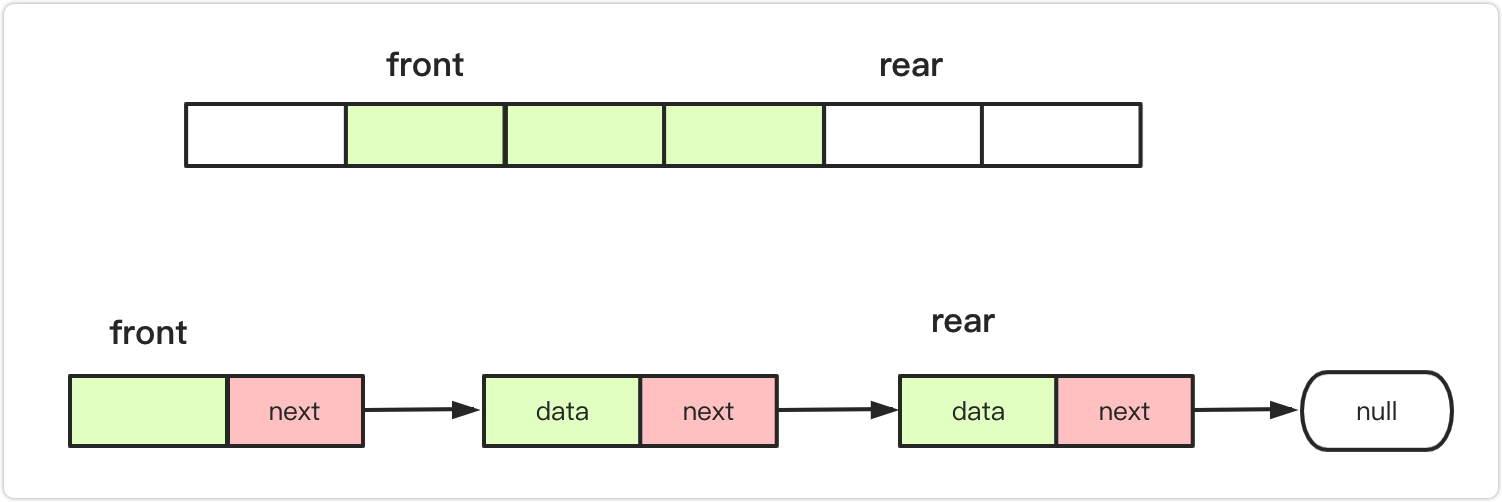• MyCircularQueue(k): 构造器，设置队列长度为 k 。
• Front: 从队首获取元素。如果队列为空，返回 -1 。
• Rear: 获取队尾元素。如果队列为空，返回 -1 。
• enQueue(value): 向循环队列插入一个元素。如果成功插入则返回真。
• deQueue(): 从循环队列中删除一个元素。如果成功删除则返回真。
• isEmpty(): 检查循环队列是否为空。
• isFull(): 检查循环队列是否已满。

### 普通队列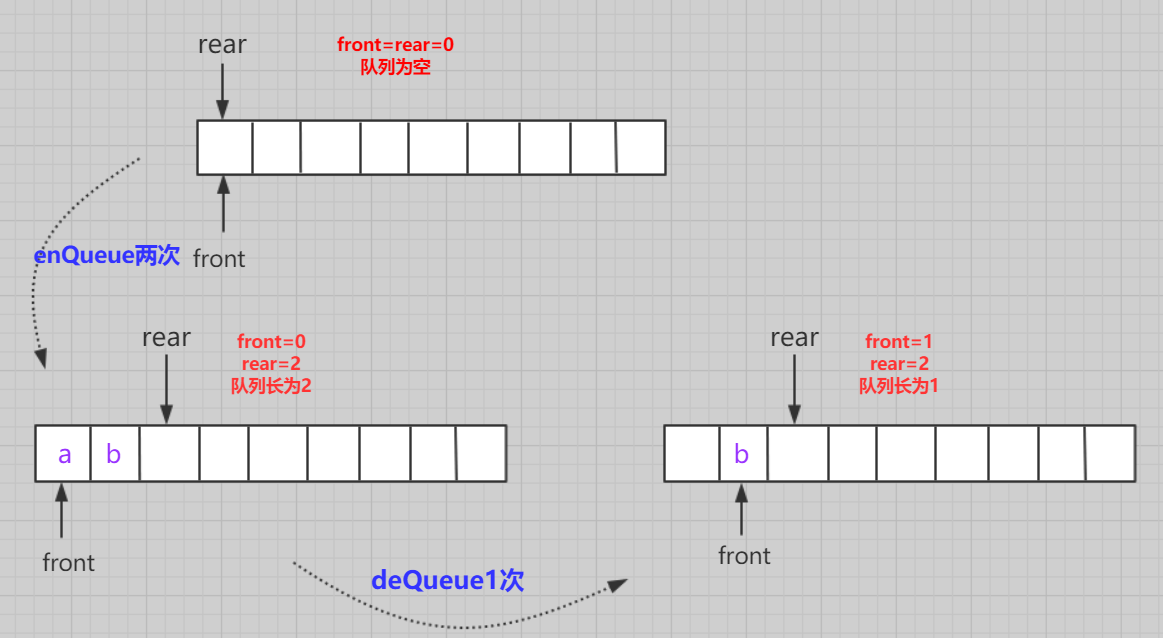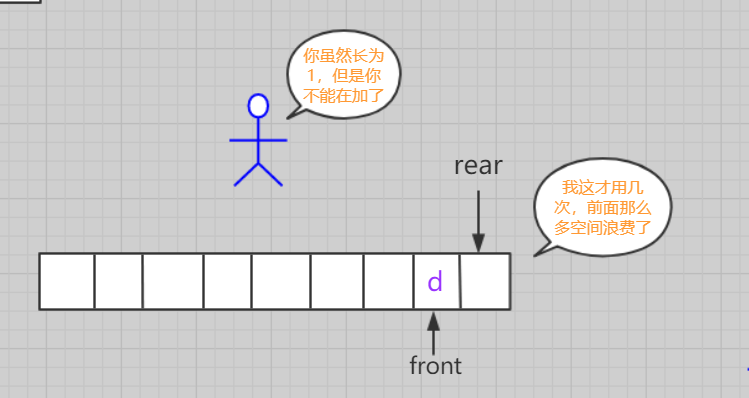### 循环队列(数组实现)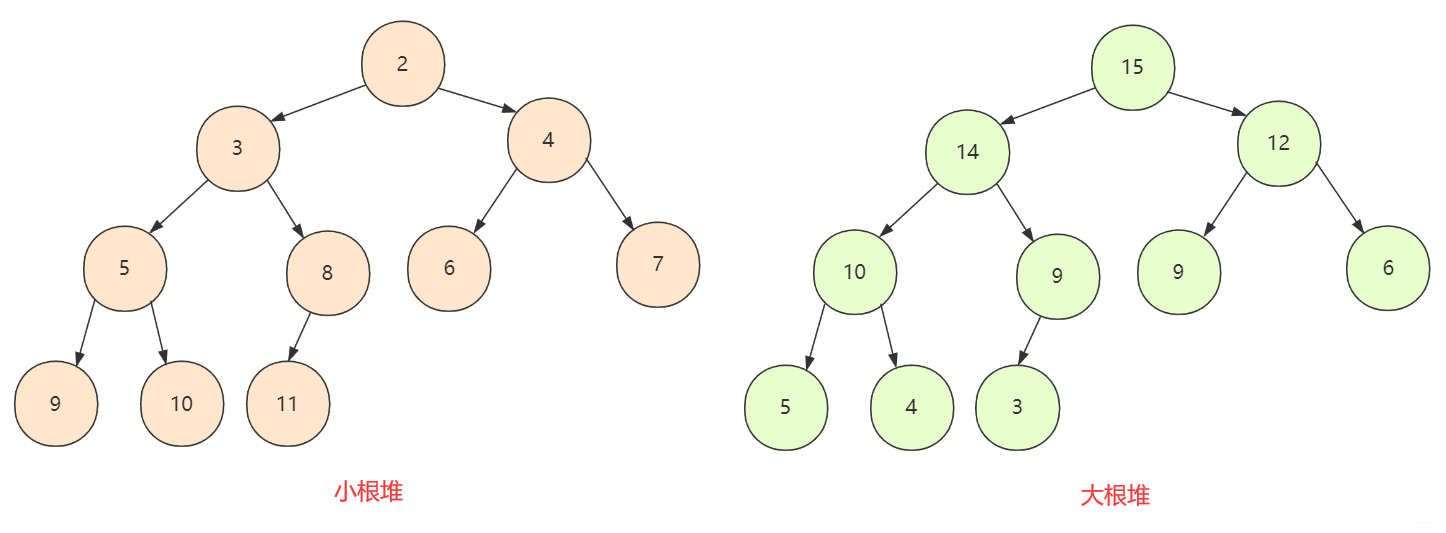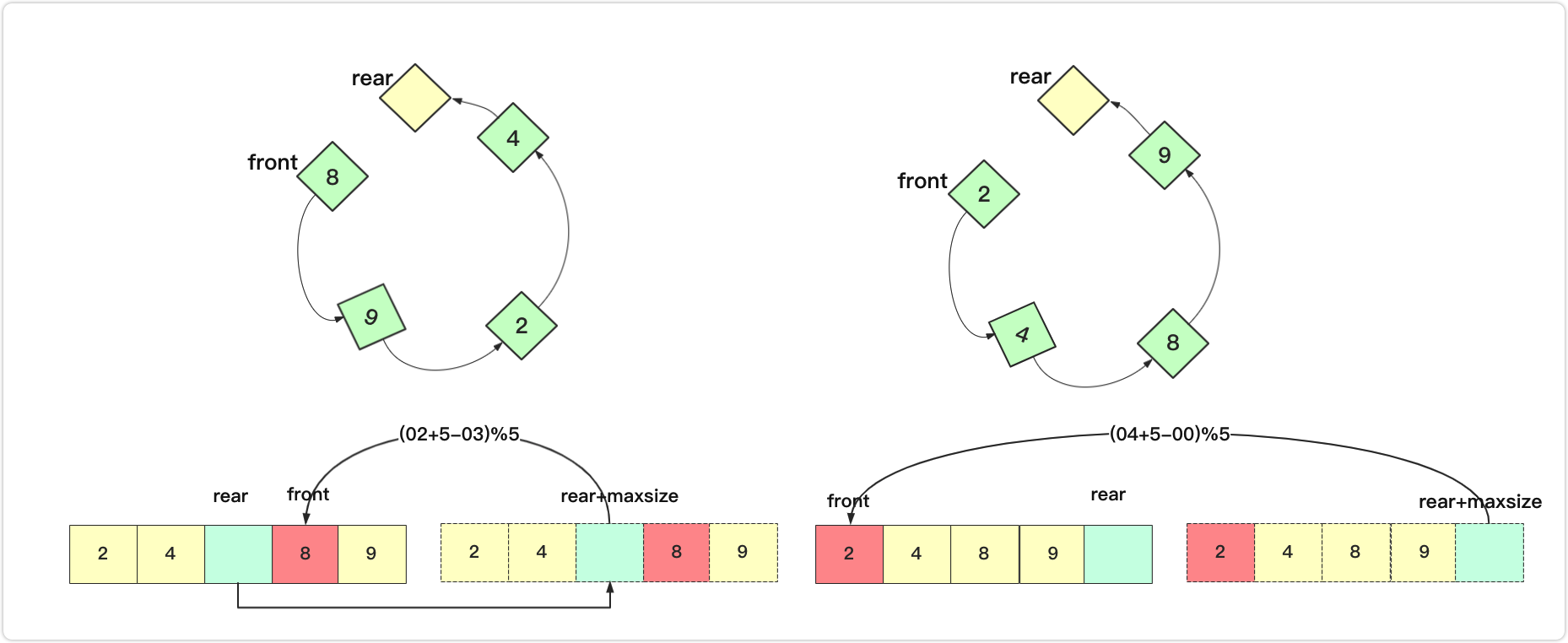``````public class MyCircularQueue {
private int data[];// 数组容器
private int front;// 头
private int rear;// 尾
private int maxsize;// 最大长度
public MyCircularQueue(int k) {
data = new int[k+1];
front = 0;
rear = 0;
maxsize = k+1;
}

public boolean enQueue(int value)  {
if (isFull())
return  false;
else {
data[rear] = value;
rear=(rear + 1) % maxsize;
}
return  true;
}

public boolean deQueue() {
if (isEmpty())
return false;
else {
front=(front+1)%maxsize;
}
return  true;
}

public int Front() {
if(isEmpty())
return -1;
return data[front];
}

public int Rear() {
if(isEmpty())
return -1;
return data[(rear-1+maxsize)%maxsize];
}

public boolean isEmpty() {
return rear == front;
}

public boolean isFull() {
return (rear + 1) % maxsize == front;
}
}
``````

### 循环队列(链表实现)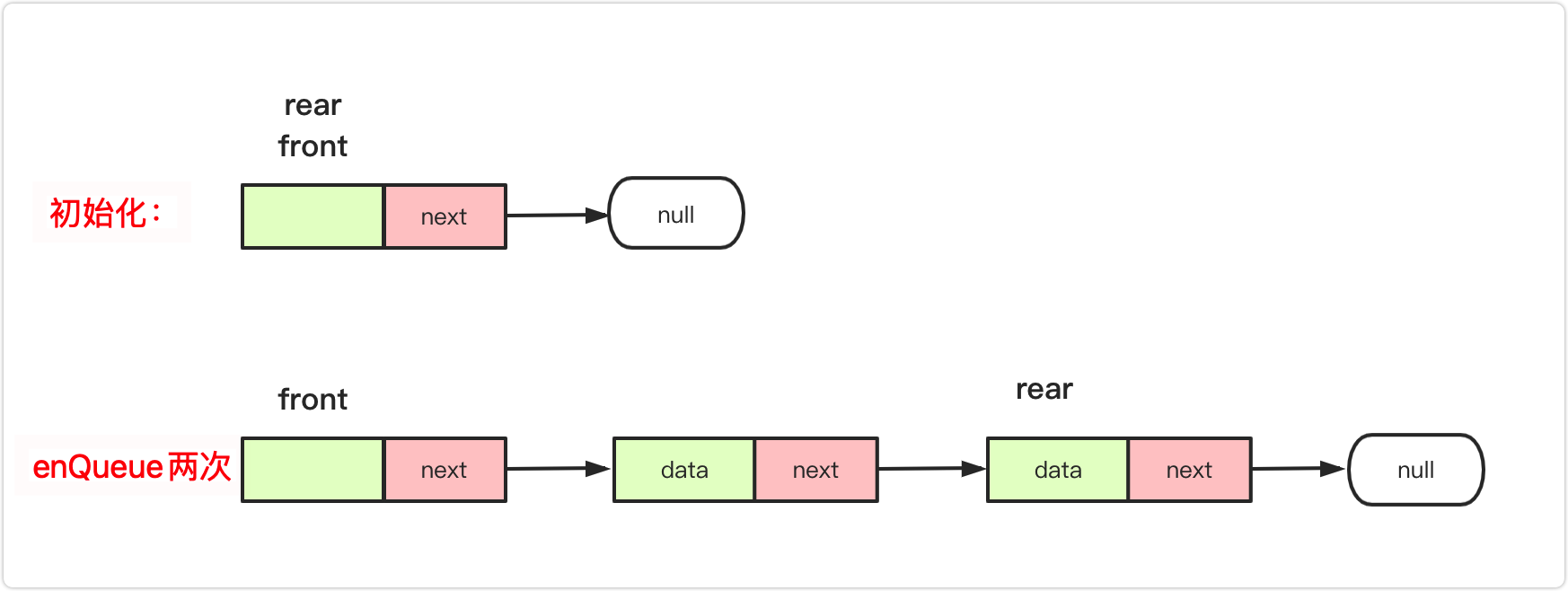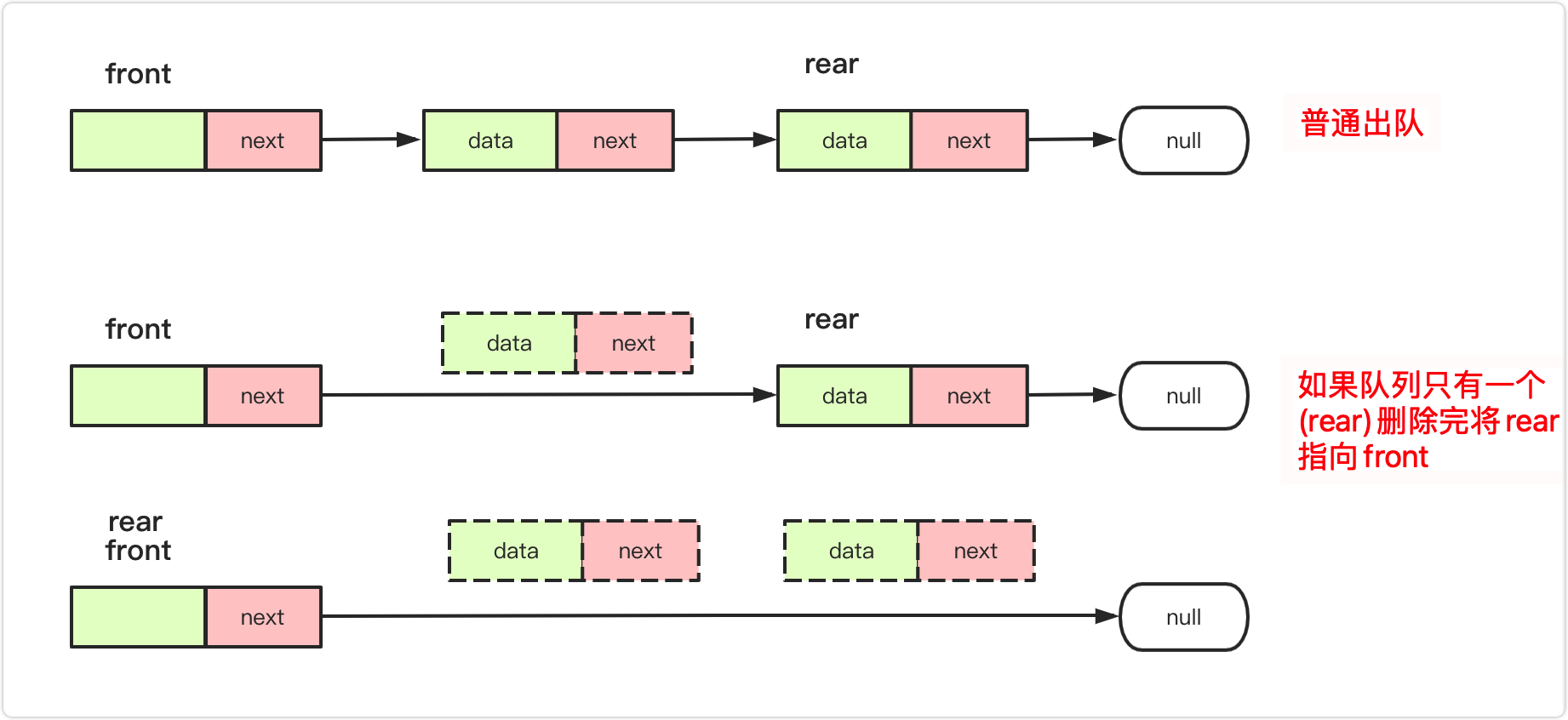``````public class MyCircularQueue{
class node {
int data;// 节点的结果
node next;// 下一个连接的节点
public node() {}
public node(int data) {
this.data = data;
}
}
node front;//相当于head 带头节点的
node rear;//相当于tail/end
int maxsize;//最大长度
int len=0;
public MyCircularQueue(int k) {
front=new node(0);
rear=front;
maxsize=k;
len=0;
}
public boolean enQueue(int value)  {
if (isFull())
return  false;
else {
node va=new node(value);
rear.next=va;
rear=va;
len++;
}
return  true;
}
public boolean deQueue() {
if (isEmpty())
return false;
else {
front.next=front.next.next;
len--;
//注意 如果被删完 需要将rear指向front
if(len==0)
rear=front;
}
return  true;
}

public int Front() {
if(isEmpty())
return -1;
return front.next.data;
}

public int Rear() {
if(isEmpty())
return -1;
return rear.data;
}

public boolean isEmpty() {
return  len==0;
//return rear == front;
}

public boolean isFull() {
return len==maxsize;
}
}
``````

### 双向队列(加餐)

• MyCircularDeque(k)：构造函数,双端队列的大小为k。
• insertFront()：将一个元素添加到双端队列头部。 如果操作成功返回 true。
• insertLast()：将一个元素添加到双端队列尾部。如果操作成功返回 true。
• deleteFront()：从双端队列头部删除一个元素。 如果操作成功返回 true。
• deleteLast()：从双端队列尾部删除一个元素。如果操作成功返回 true。
• getFront()：从双端队列头部获得一个元素。如果双端队列为空，返回 -1。
• getRear()：获得双端队列的最后一个元素。 如果双端队列为空，返回 -1。
• isEmpty()：检查双端队列是否为空。
• isFull()：检查双端队列是否满了。

``````public class MyCircularDeque {
private int data[];// 数组容器
private int front;// 头
private int rear;// 尾
private int maxsize;// 最大长度
/*初始化 最大大小为k */
public MyCircularDeque(int k) {
data = new int[k+1];
front = 0;
rear = 0;
maxsize = k+1;
}

/** 头部插入 */
public boolean insertFront(int value) {
if(isFull())
return false;
else {
front=(front+maxsize-1)%maxsize;
data[front]=value;
}
return  true;
}

/** 尾部插入 */
public boolean insertLast(int value) {
if(isFull())
return  false;
else{
data[rear]=value;
rear=(rear+1)%maxsize;
}
return  true;
}

/** 正常头部删除 */
public boolean deleteFront() {
if (isEmpty())
return false;
else {
front=(front+1)%maxsize;
}
return  true;
}

/** 尾部删除 */
public boolean deleteLast() {
if(isEmpty())
return false;
else {
rear=(rear+maxsize-1)%maxsize;
}
return true;
}

/** Get the front item  */
public int getFront() {
if(isEmpty())
return -1;
return data[front];
}

/** Get the last item from the deque. */
public int getRear() {
if(isEmpty())
return -1;
return  data[(rear-1+maxsize)%maxsize];
}

/** Checks whether the circular deque is empty or not. */
public boolean isEmpty() {
return front==rear;
}

/** Checks whether the circular deque is full or not. */
public boolean isFull() {
return (rear+1)%maxsize==front;
}
}
``````

### 总结

【版权声明】本文为华为云社区用户原创内容，转载时必须标注文章的来源（华为云社区），文章链接，文章作者等基本信息，否则作者和本社区有权追究责任。如果您发现本社区中有涉嫌抄袭的内容，欢迎发送邮件至：huaweicloud.bbs@huawei.com进行举报，并提供相关证据，一经查实，本社区将立刻删除涉嫌侵权内容。